Share

# RD Sharma solutions for Class 10 Mathematics chapter 8 - Circles [Latest edition]

Course
Textbook page

#### Chapters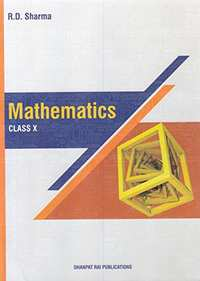## Chapter 8: Circles

OthersEx. 8.1Ex. 8.2

#### RD Sharma solutions for Class 10 Mathematics Chapter 8 Circles [Pages 34 - 37]

Page 37

In Fig. 7, two equal circles, with centres O and O’, touch each other at X. OO’ produced meets the circle with centre O’ at A. AC is tangent to the circle with centre O, at the point C. O’D is perpendicular to AC. Find the value of (DO')/(CO')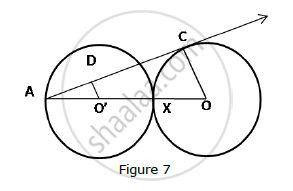Page 34

In the fig. ABC is right triangle right angled at B such that BC = 6cm and AB = 8cm. Find the radius of its in circle.

Page 34

If ΔABC is isosceles with AB = AC and C (0, 2) is the in circle of the ΔABC touching BC at L, prove that L, bisects BC.

Page 35

In fig.. O is the center of the circle and BCD is tangent to it at C. Prove that ∠BAC +
∠ACD = 90°

Page 35

In the given figure, PO⊥QO. The tangents to the circle at P and Q intersect at a point T. Prove that PQ and OT are right bisector of each other.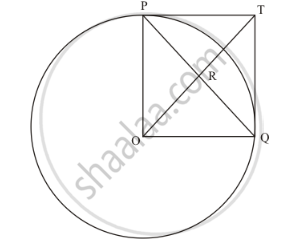Page 35

In the fig two tangents AB and AC are drawn to a circle O such that ∠BAC = 120°. Prove that OA = 2AB.

Page 36

In the given figure, BC is a tangent to the circle with centre O. OE bisects AP. Prove that ΔAEO~Δ ABC.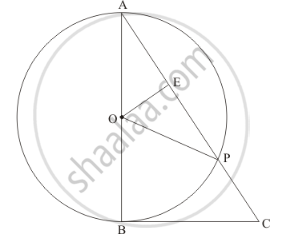Page 36

The lengths of three consecutive sides of a quadrilateral circumscribing a circle are 4cm,5cm and 7cm respectively. Determine the length of fourth side.

Page 36

In fig common tangents PQ and RS to two circles intersect at A. Prove that PQ = RS.

Page 37

In figure OQ : PQ = 3 : 4 and perimeter of ΔPDQ = 60cm. determine PQ, QR and OP.

#### RD Sharma solutions for Class 10 Mathematics Chapter 8 CirclesExercise 8.1 [Page 5]

Ex. 8.1 | Q 1.1 | Page 5

Fill in the blank:

The common point of a tangent to a circle and the circle is called ____

Ex. 8.1 | Q 1.2 | Page 5

Fill in the blank:

A circle can have __________ parallel tangents at the most.

Ex. 8.1 | Q 1.3 | Page 5

Fill in the blank:

A tangent to a circle intersects it in _______ point (s).

Ex. 8.1 | Q 1.4 | Page 5

Fill in the blank:

A line intersecting a circle in two points is called a __________.

Ex. 8.1 | Q 1.5 | Page 5

Fill in the blank

The angle between tangent at a point on a circle and the radius through the point is ........

Ex. 8.1 | Q 2 | Page 5

How many tangents can a circle have?

Ex. 8.1 | Q 3 | Page 5

O is the center of a circle of radius 8cm. The tangent at a point A on the circle cuts a line through O at B such that AB = 15 cm. Find OB

Ex. 8.1 | Q 4 | Page 5

If the tangent at point P to the circle with center O cuts a line through O at Q such that PQ= 24cm and OQ = 25 cm. Find the radius of circle

#### RD Sharma solutions for Class 10 Mathematics Chapter 8 CirclesExercise 8.2 [Pages 33 - 42]

Ex. 8.2 | Q 1 | Page 33

If PT is a tangent at T to a circle whose center is O and OP = 17 cm, OT = 8 cm. Find the length of tangent segment PT.

Ex. 8.2 | Q 2 | Page 33

Find the length of a tangent drawn to a circle with radius 5cm, from a point 13 cm from the center of the circle.

Ex. 8.2 | Q 3 | Page 33

A point P is 26 cm away from O of circle and the length PT of the tangent drawn from P to the circle is 10 cm. Find the radius of the circle.

Ex. 8.2 | Q 4 | Page 33

If from any point on the common chord of two intersecting circles, tangents be drawn to circles, prove that they are equal.

Ex. 8.2 | Q 5 | Page 33

If the quadrilateral sides touch the circle prove that sum of pair of opposite sides is equal to the sum of other pair.

Ex. 8.2 | Q 6 | Page 33

Out of the two concentric circles , the radius of the outer circle is 5 cm and the chord AC of length 8 cm is a tangent to the inner circle . Find the radius of the inner circle .

Ex. 8.2 | Q 7 | Page 33

A chord PQ of a circle is parallel to the tangent drawn at a point R of the circle . Prove that R bisects the arc PRQ.

Ex. 8.2 | Q 8 | Page 33

Prove that a diameter AB of a circle bisects all those chords which are parallel to the tangent at that point A .

Ex. 8.2 | Q 9 | Page 33

If AB, AC, PQ are tangents in Fig. and AB = 5cm find the perimeter of ΔAPQ.

Ex. 8.2 | Q 10 | Page 34

Prove that the intercept of a tangent between two parallel tangents to a circle subtends a right angle at center.

Ex. 8.2 | Q 11 | Page 34

In Fig below, PQ is tangent at point R of the circle with center O. If ∠TRQ = 30°. Find
∠PRS.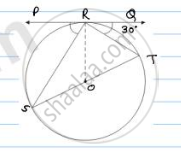Ex. 8.2 | Q 12 | Page 34

If PA and PB are tangents from an outside point P. such that PA = 10 cm and ∠APB = 60°. Find the length of chord AB.

Ex. 8.2 | Q 13 | Page 34

In a right triangle ABC in which  $\angle$B= 900 , a circle is drawn with AB as diameter intersecting the hypotenuse AC at P . Prove that the tangent to the circle at P bisects BC.

Ex. 8.2 | Q 14 | Page 34

From an external point P, tangents PA and PB are drawn to the circle with centre O. If CD is the tangent to the circle at point E and PA = 14 cm. Find the perimeter of ABCD.

Ex. 8.2 | Q 15 | Page 34

In the given figure, ABC is a right triangle right-angled at B such that BC = 6 cm and AB = 8 cm. Find the radius of its incircle.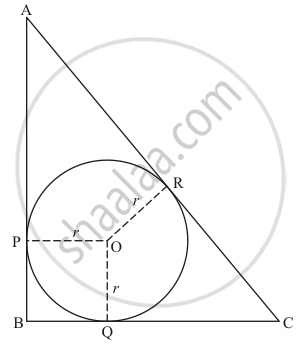Ex. 8.2 | Q 16 | Page 35

Prove that the tangent drawn at the mid-point of an arc of a circle is parallel to the chord joining the end points of the arc.

Ex. 8.2 | Q 17 | Page 35

From a point P, two tangents PA and PB are drawn to a circle with center O. If OP =
diameter of the circle shows that ΔAPB is equilateral.

Ex. 8.2 | Q 18 | Page 35

Two tangent segments PA and PB are drawn to a circle with center O such that ∠APB =120°. Prove that OP = 2AP

Ex. 8.2 | Q 19 | Page 35

If Δ ABC is isosceles with AB = AC and C (O, r) is the incircle of the ΔABC touching BC at L,prove that L bisects BC.

Ex. 8.2 | Q 20 | Page 35

AB is a diameter  and AC is a chord of a circle with centre such that $\angle BAC = {30}^o$ . The tangent at C intersects AB  at a point D . Prove that BC = BD.

Ex. 8.2 | Q 21 | Page 35

In fig. a circle touches all the four sides of quadrilateral ABCD with AB = 6cm, BC = 7cm, CD = 4cm. Find AD.

Ex. 8.2 | Q 22 | Page 35

Prove that the perpendicular at the point of contact to the tangent to a circle passes through the centre

Ex. 8.2 | Q 23 | Page 35

Two circles touch externally at a point P. from a point T on the tangent at P, tangents TQ and TR are drawn to the circles with points of contact Q and E respectively. Prove that TQ = TR.

Ex. 8.2 | Q 24 | Page 35

is a point at a distance 13 cm from the centre O of a circle  of radius  5 cm . AP and AQ  are the tangents to the circle at P and Q . If a tangent BC is drawn at a point R lying on the minor arc PQ to intersect  AP at  and AQ at C , find the perimeter of the $∆$ABC .

Ex. 8.2 | Q 25 | Page 35

In the fig. a circle is inscribed in a quadrilateral ABCD in which ∠B = 90° if AD = 23cm,
AB = 29cm and DS = 5cm, find the radius of the circle.

Ex. 8.2 | Q 26 | Page 35

In fig. there are two concentric circles with Centre O of radii 5cm and 3cm. From an
external point P, tangents PA and PB are drawn to these circles if AP = 12cm, find the
tangent length of BP.

Ex. 8.2 | Q 27 | Page 35

In the given figure, AB is a chord of length 16 cm of a circle of radius 10 cm. The tangents at A and B intersect at a point P. Find the length of PA.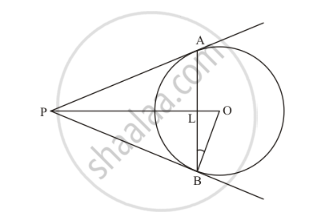Ex. 8.2 | Q 28 | Page 37

In figure PA and PB are tangents from an external point P to the circle with centre O. LN touches the circle at M. Prove that PL + LM = PN + MN

Ex. 8.2 | Q 29.1 | Page 37

In the given figure, BDC is a tangent to the given circle at point D such that BD = 30 cm and CD = 7 cm. The other tangents BE and CF are drawn respectively from B and C to the circle and meet when produced at A making BAC a right angle triangle. Calculate (i) AF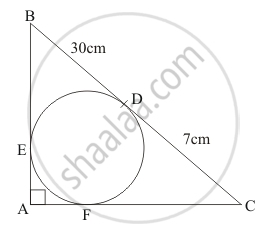Ex. 8.2 | Q 29.2 | Page 37

In the given figure, BDC is a tangent to the given circle at point D such that BD = 30 cm and CD = 7 cm. The other tangents BE and CF are drawn respectively from B and C to the circle and meet when produced at A making BAC a right angle triangle. Calculate (ii) radius of the circle.Ex. 8.2 | Q 30 | Page 37

If $d_1 , d_2 ( d_2 > d_1 )$ be the diameters of two concentric circle s and c be the length of a chord of a circle which is tangent to the other circle , prove that${d_2}^2 = c^2 + {d_1}^2$.

Ex. 8.2 | Q 31 | Page 37

In the given figure, tangents PQ and PR are drawn from an external point to a circle with centre O, such that$\angle RPQ = 30 ^o$. A chord RS is drawn parallel to the tangent PQ. Find$\angle RQS$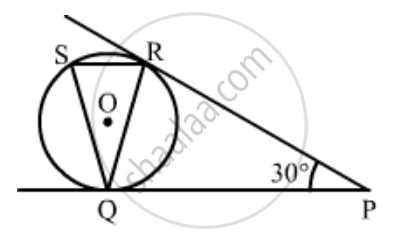Ex. 8.2 | Q 32 | Page 37

From an external point P , tangents PA PB are drawn to a circle with centre O   . If  $\angle PAB = {50}^o$ , then find  $\angle AOB$

Ex. 8.2 | Q 33 | Page 38

In the given figure, two tangents AB and AC are drawn to a circle with centre O such that ∠BAC = 120°. Prove that OA = 2AB.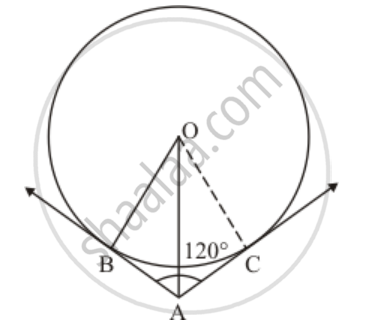Ex. 8.2 | Q 34 | Page 38

The length of three concesutive sides of a quadrilateral circumscribing a circle are 4 cm, 5 cm, and 7 cm respectively. Determine the length of the fourth side.

Ex. 8.2 | Q 35 | Page 38

The common tangents AB and CD to two circles with centres O and O' intersect at between their centres . Prove that the  points and O' are collinear .

Ex. 8.2 | Q 36 | Page 38

In the given figure, common tangents PQ and RS to two circles intersect at A. Prove that PQ = RS.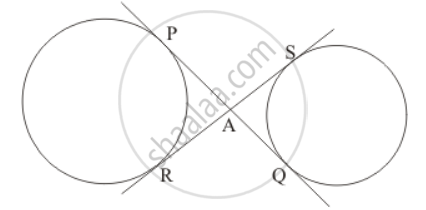Ex. 8.2 | Q 37 | Page 38

Two concentric circles are of diameters 30 cm and 18 cm. Find the length of the chord of the larger circle which touches the smaller circle.

Ex. 8.2 | Q 38 | Page 38

AB and CD are common tangents to two circles of equal radii. Prove that AB = CD.

Ex. 8.2 | Q 39 | Page 38

A triangle PQR is drawn to circumscribe a circle of radius 8 cm such that the segments QT and TR, into which QR is divided by the point of contact T, are of lengths 14 cm and 16 cm respectively. If area of ∆PQR is 336 cm2, find the sides PQ and PR.

Ex. 8.2 | Q 40 | Page 39

In Fig . 10.69, the tangent at a point of a circle and a diameter AB when extended intersect at P . If $\angle$PCA =1100, find $\angle$ CBA

Ex. 8.2 | Q 41 | Page 39

AB is a chord of a circle with centre O , AOC  is a diameter and AT is the tangent at A as shown in Fig . 10.70. Prove that $\angle$BAT = $\angle$ ACB

Ex. 8.2 | Q 42 | Page 39

In the given figure, a ∆ABC is drawn to circumscribe a circle of radius 4 cm such that the segments BD and DC are of lengths 8 cm and 6 cm respectively. Find the lengths of sides AB and AC, when area of ∆ABC is 84 cm2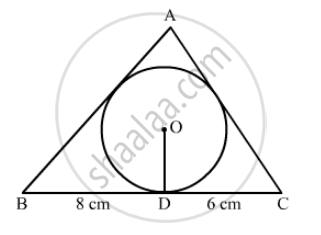Ex. 8.2 | Q 43 | Page 39

In the given figure, AB is a diameter of a circle with centre O and AT is a tangent. If $\angle$ AOQ = 58º, find  $\angle$ ATQ.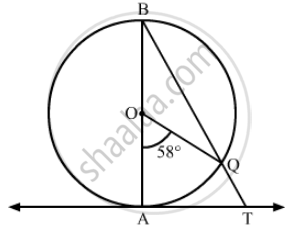Ex. 8.2 | Q 44 | Page 40

In the given figure, OQ : PQ = 3.4 and perimeter of Δ POQ = 60 cm. Determine PQ, QR and OP.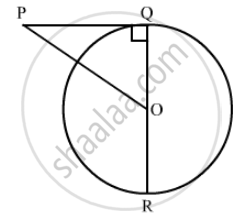Ex. 8.2 | Q 45 | Page 40

Equal circles with centres O and O' touch each other at X. OO' produced to meet a circle with centre O', at A. AC is a tangent to the circle whose centre is O. O'D is perpendicular to AC. Find the value of$\frac{DO'}{CO}$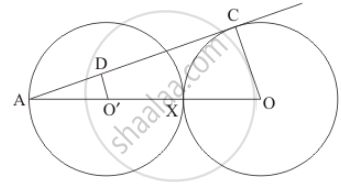Ex. 8.2 | Q 46 | Page 40

In the given figure, BC is a tangent to the circle with centre OOE bisects AP. Prove that ΔAEO ∼ Δ ABC.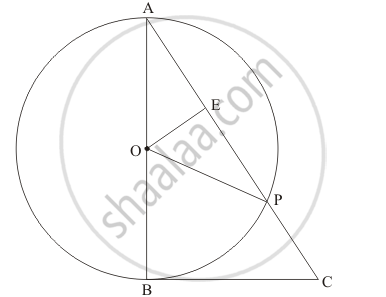Ex. 8.2 | Q 47 | Page 40

In the given figure, PO $\perp$  QO. The tangents to the circle at P and Q intersect at a point T. Prove that PQ and OTare right bisector of each other.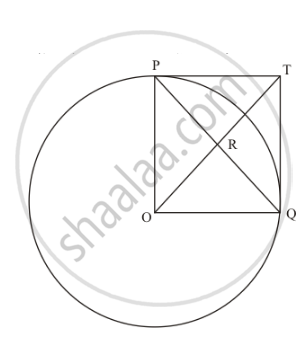Ex. 8.2 | Q 48 | Page 41

In the given figure, O is the centre of the circle and BCD is tangent to it at C. Prove that ∠BAC + ∠ACD = 90°.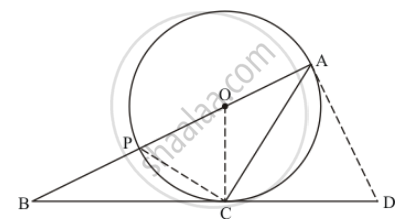Ex. 8.2 | Q 49 | Page 41

Prove that the centre of a circle touching two intersecting lines lies on the angle bisector of the lines .

Ex. 8.2 | Q 50 | Page 41

In Fig. 8.78, there are two concentric circles with centre O. PRT and PQS are tangents to the inner circle from a point P lying on the outer circle. If PR = 5 cm, find the length of PS.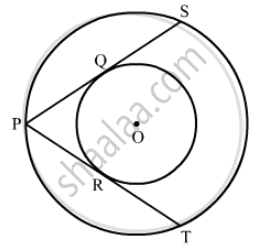Ex. 8.2 | Q 51 | Page 41

In Fig. 8.79, PQ is a tangent from an external point P to a circle with centre O and OP cuts the circle at T and QOR is a diameter. If ∠POR = 130° and S is a point on the circle, find ∠1 + ∠2.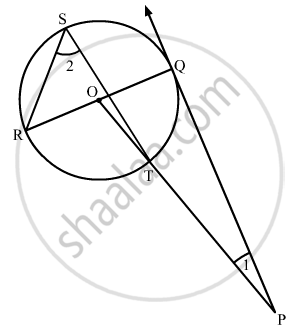Ex. 8.2 | Q 52 | Page 42

In the given figure, PA and PB are tangents to the circle from an external point P. CD is another tangent touching the circle at Q. If PA = 12 cm, QC = QD = 3 cm, then find PC + PD.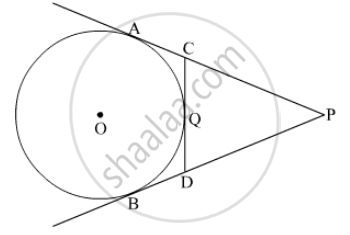## Chapter 8: Circles

OthersEx. 8.1Ex. 8.2## RD Sharma solutions for Class 10 Mathematics chapter 8 - Circles

RD Sharma solutions for Class 10 Mathematics chapter 8 (Circles) include all questions with solution and detail explanation. This will clear students doubts about any question and improve application skills while preparing for board exams. The detailed, step-by-step solutions will help you understand the concepts better and clear your confusions, if any. Shaalaa.com has the CBSE Class 10 Mathematics solutions in a manner that help students grasp basic concepts better and faster.

Further, we at Shaalaa.com provide such solutions so that students can prepare for written exams. RD Sharma textbook solutions can be a core help for self-study and acts as a perfect self-help guidance for students.

Concepts covered in Class 10 Mathematics chapter 8 Circles are Theorem - Converse of Tangent at Any Point to the Circle is Perpendicular to the Radius, Number of Tangents from a Point to a Circle, Inscribed Angle Theorem, Intercepted Arc, Inscribed Angle, Property of Sum of Measures of Arcs, Tangent Segment Theorem, Converse of Tangent Theorem, Circles passing through one, two, three points, Theorem of External Division of Chords, Theorem of Internal Division of Chords, Converse of Theorem of the Angle Between Tangent and Secant, Theorem of Angle Between Tangent and Secant, Converse of Cyclic Quadrilateral Theorem, Corollary of Cyclic Quadrilateral Theorem, Theorem of Cyclic Quadrilateral, Corollaries of Inscribed Angle Theorem, Angle Subtended by the Arc to the Point on the Circle, Angle Subtended by the Arc to the Centre, Introduction to an Arc, Touching Circles, Number of Tangents from a Point on a Circle, Tangent to a Circle, Tangents and Its Properties, Tangent Properties - If Two Circles Touch, the Point of Contact Lies on the Straight Line Joining Their Centers, Cyclic Properties, Tangent - Secant Theorem, Cyclic Quadrilateral, Introduction to Circles, Circles Examples and Solutions, Introduction to Circles, Tangent to a Circle, Number of Tangents from a Point on a Circle.

Using RD Sharma Class 10 solutions Circles exercise by students are an easy way to prepare for the exams, as they involve solutions arranged chapter-wise also page wise. The questions involved in RD Sharma Solutions are important questions that can be asked in the final exam. Maximum students of CBSE Class 10 prefer RD Sharma Textbook Solutions to score more in exam.

Get the free view of chapter 8 Circles Class 10 extra questions for Class 10 Mathematics and can use Shaalaa.com to keep it handy for your exam preparation

S